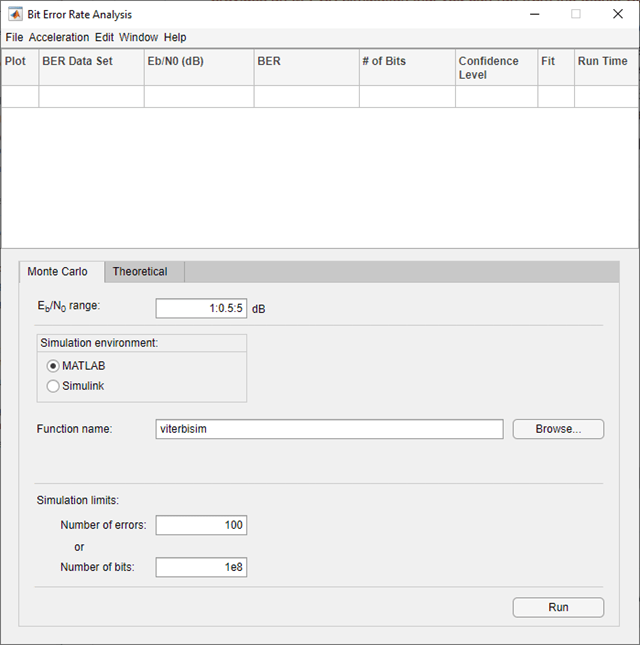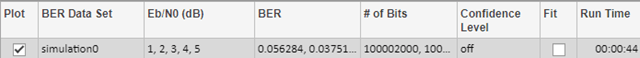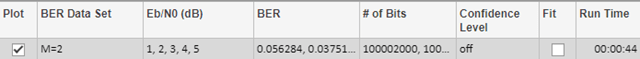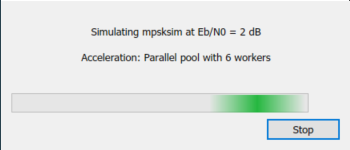# Bit Error Rate Analysis

Analyze BER performance of communications systems

## Description

The Bit Error Rate Analysis app calculates the bit error rate (BER) as a function of the energy per bit to noise power spectral density ratio (Eb/N0). Using this app, you can:

• Generate BER data for a communications system and analyze performance using:

• Monte Carlo simulations of MATLAB® functions and Simulink® models.

• Theoretical closed-form expressions for selected types of communications systems.

• Run systems contained in MATLAB simulation functions or Simulink models. After you create a function or model that simulates the system, the Bit Error Rate Analysis app iterates over your choice of Eb/N0 values and collects the results.

• Plot one or more BER data sets on a single set of axes. You can graphically compare simulation data with theoretical results or simulation data from a series of communications system models.

• Fit a curve to a set of simulation data.

• Plot confidence levels of simulation data.

• Send BER data to the MATLAB workspace or to a file for further processing.

## Open the Bit Error Rate Analysis App

• MATLAB Toolstrip: On the Apps tab, under Signal Processing and Communications, click the app icon.

• MATLAB command prompt: Enter `bertool`.

## Examples

expand all

Generate a theoretical estimate of BER performance for a 16-QAM link in AWGN.

Open the Bit Error Rate Analysis app.

`bertool`On the Theoretical tab, set these parameters to the specified values: Eb/N0 range to `0:10`, Modulation type to `QAM`, and Modulation order to `16`.

Plot the BER curve by clicking .Simulate the BER by using a custom MATLAB function. By default, the app uses the `viterbisim.m` simulation.

Open the Bit Error Rate Analysis app.

`bertool`

On the Monte Carlo tab, set the Eb/N0 range parameter to `1:.5:6`. Run the simulation and plot the estimated BER values by clicking Run.

On the Theoretical tab, set Eb/N0 range to `1:6` and set Modulation order to `4`. Enable convolutional coding by selecting . Click Plot to add the theoretical upper bound of the BER curve to the plot.Add code to the simulation function template given in the Template for Simulation Function topic to run in the Monte Carlo tab of the Bit Error Rate Analysis.

Prepare Function

Copy the template from the Template for Simulation Function topic into a new MATLAB file in the MATLAB Editor. Save the file in a folder on your MATLAB path, using the file name `bertool_simfcn`.

Place lines of code that initialize parameters or create objects used in the simulation in the template section marked ```Set up initial parameters```. This code maps simulation variables to the template input arguments. For example, `snr` maps to `EbNo`.

```% Set up initial parameters. siglen = 1000; % Number of bits in each trial M = 2; % DBPSK is binary snr = EbNo; % Because of binary modulation % Create an ErrorRate calculator System object to compare % decoded symbols to the original transmitted symbols. errorCalc = comm.ErrorRate;```

Place the code for the core simulation tasks in the template section marked `Proceed with simulation`. This code includes the core simulation tasks, after all setup work has been performed.

``` msg = randi([0,M-1],siglen,1); % Generate message sequence txsig = dpskmod(msg,M); % Modulate hChan.SignalPower = ... % Calculate and assign signal power (txsig'*txsig)/length(txsig); rxsig = awgn(txsig,snr,'measured'); % Add noise decodmsg = dpskdemod(rxsig,M); % Demodulate berVec = errorCalc(msg,decodmsg); % Calculate BER totErr = totErr + berVec(2); numBits = numBits + berVec(3); ```

After you insert these two code sections into the template, the `bertool_simfcn` function is compatible with the Bit Error Rate Analysis app. The resulting code resembles this code segment.

```function [ber,numBits] = bertool_simfcn(EbNo,maxNumErrs,maxNumBits,varargin) % % See also BERTOOL and VITERBISIM. % Copyright 2020 The MathWorks, Inc. % Initialize variables related to exit criteria. totErr = 0; % Number of errors observed numBits = 0; % Number of bits processed % --- Set up the simulation parameters. --- % --- INSERT YOUR CODE HERE. % Set up initial parameters. siglen = 1000; % Number of bits in each trial M = 2; % DBPSK is binary. snr = EbNo; % Because of binary modulation % Create an ErrorRate calculator System object to compare % decoded symbols to the original transmitted symbols. errorCalc = comm.ErrorRate; % Simulate until the number of errors exceeds maxNumErrs % or the number of bits processed exceeds maxNumBits. while((totErr < maxNumErrs) && (numBits < maxNumBits)) % Check if the user clicked the Stop button of BERTool. if isBERToolSimulationStopped(varargin{:}) break end % --- Proceed with the simulation. % --- Update totErr and numBits. % --- INSERT YOUR CODE HERE. msg = randi([0,M-1],siglen,1); % Generate message sequence txsig = dpskmod(msg,M); % Modulate hChan.SignalPower = ... % Calculate and assign signal power (txsig'*txsig)/length(txsig); rxsig = awgn(txsig,snr,'measured'); % Add noise decodmsg = dpskdemod(rxsig,M); % Demodulate berVec = errorCalc(msg,decodmsg); % Calculate BER totErr = totErr + berVec(2); numBits = numBits + berVec(3); end % End of loop % Compute the BER. ber = totErr/numBits;```

The function has inputs to specify the app and scalar quantities for `EbNo`, `maxNumErrs`, and `maxNumBits` that are provided by the app. The Bit Error Rate Analysis app is an input because the function monitors and responds to the stop command in the app. The `bertool_simfcn` function excludes code related to plotting, curve fitting, and confidence intervals because the Bit Error Rate Analysis app enables you to do similar tasks interactively without writing code.

Use Prepared Function

Run `bertool_simfcn` in the Bit Error Rate Analysis app.

Open the Bit Error Rate Analysis app, and then select the Monte Carlo tab.

Set these parameters to the specified values: Eb/N0 range to `0:10`, Simulation environment to `MATLAB`, Function name to `bertool_simfcn`, Number of errors to `5`, and Number of bits to `1e8`.Click .

The Bit Error Rate Analysis app computes the results and then plots them. In this case, the results do not appear to fall along a smooth curve because the simulation required only five errors for each value in `EbNo`.Fit a curve to the series of points in the BER Figure window, by selecting the Fit parameter in the data viewer.The Bit Error Rate Analysis app plots the fitted curve, as shown in this figure.Use the Bit Error Rate Analysis app to compute the BER as a function of ${\mathit{E}}_{\mathrm{b}}/{\mathit{N}}_{0}$. The app analyzes performance with either Monte Carlo simulations of MATLAB® functions and Simulink® models or theoretical closed-form expressions for selected types of communications systems. The code in the mpsksim.m function provides an M-PSK simulation that you can run from the Monte Carlo tab of the app.

Open the Bit Error Rate Analysis app from the Apps tab or by running the `bertool` function in the MATLAB command window.On the Monte Carlo tab, set the ${\mathbit{E}}_{\mathbf{b}}/{\mathbit{N}}_{0}$ range parameter to `1:1:5` and the Function name parameter to `mpsksim`.Open the `mpsksim` function for editing, set `M=2`, and save the changed file.

Run the `mpsksim.m` function as configured by clicking Run on the Monte Carlo tab in the app.

After the app simulates the set of ${\mathit{E}}_{\mathrm{b}}/{\mathit{N}}_{0}$ points, update the name of the BER data set results by selecting `simulation0` in the BER Data Set field and typing `M=2` to rename the set of results. The legend on the BER figure updates the label to `M=2`.Update the value for `M` in the `mpsksim` function, repeating this process for `M` = `4`, `8`, and `16`. For example, these figures of the Bit Error Rate Analysis app and BER Figure window show results for varying `M` values.Parallel SNR Sweep Using Bit Error Rate Analysis App

The default configuration for the Monte Carlo processing of the Bit Error Rate Analysis app automatically uses parallel pool processing to process individual ${\mathit{E}}_{\mathrm{b}}/{\mathit{N}}_{0}$ points when you have the Parallel Computing Toolbox™ software but for the processing of your simulation code:Use a Simulink simulation model to run in the Monte Carlo tab of the Bit Error Rate Analysis app. Compare the BER performance of the Simulink simulation results with theoretical BER results.

Prepare Model

Open the model by entering `doc_bpsk` at the MATLAB command prompt.

`doc_bpsk`Initialize parameters in the MATLAB workspace to avoid using undefined variables as block parameters.

```EbNo = 0; maxNumErrs = 100; maxNumBits = 1e8; ```

Ensure that the Bit Error Rate Analysis app uses the correct amount of noise each time it runs the simulation, by opening the dialog box for the AWGN Channel block and verifying that the Es/No parameter is set to `EbNo`.

Note

For BPSK modulation, Es/N0 is equivalent to Eb/N0.

Ensure that the Bit Error Rate Analysis app uses the correct stopping criteria for each iteration by:

• Opening the dialog box for the Error Rate Calculation block and verifying that Target number of errors is set to `maxNumErrs` and that Maximum number of symbols is set to `maxNumBits`.

• Verifying that the simulation stop time is set to `Inf`.

Enable the Bit Error Rate Analysis app to access the BER results that the Error Rate Calculation block computes, by ensuring that the BER variable name parameter in the app matches the Variable name parameter set in the To Workspace (Simulink) block that connects to the output of the Error Rate Calculation block.

Tip

Select the To Workspace block from the DSP System Toolbox™ / Sinks sublibrary. For more information, see To Workspace Block Configuration for Communications System Simulations.

Use Prepared Model

Run the `doc_bpsk` model in the Bit Error Rate Analysis app.

Open the Bit Error Rate Analysis app, and then select the Monte Carlo tab.

Set these parameters to the specified values: Eb/N0 range to `0:9`, Simulation environment to `Simulink`, Function name to `doc_bpsk`, Number of errors to `100`, and Number of bits to `1e8`.Click .

The Bit Error Rate Analysis app computes the results and then plots them.Compare these simulation results with the theoretical results, by clicking the Theoretical tab in the Bit Error Rate Analysis app and setting Eb/N0 range to `0:9`.Click .

The Bit Error Rate Analysis app plots the theoretical curve in the BER Figure window along with the earlier simulation results.## Parameters

Theoretical

Range of Eb/N0 values over which the BER is evaluated, specified as a scalar or vector. Units are in dB.

Example: `5:10` specifies the evaluation of Eb/N0 values over the range [5, 10] at 1 dB increments.

Type of channel over which the BER is evaluated, specified as `AWGN`, `Rayleigh`, or `Rician`. The `Rayleigh` and `Rician` options correspond to flat fading channels.

Modulation type of the communications link, specified as `PSK`, `DPSK`, `OQPSK`, `PAM`, `QAM`, `FSK`, `MSK`, or `CPFSK`.

Modulation order of the communications link, specified as `2`, `4`, `8`, `16`, `32`, or `64`.

Select this parameter to enable differential encoding of the input data.

Correlation coefficient, specified as a real scalar in the range [-1, 1].

#### Dependencies

To enable this parameter, set Modulation type to `FSK`.

Modulation index, specified as a positive real scalar.

#### Dependencies

To enable this parameter, set Modulation type to `CPFSK`.

• Select this parameter to enable coherent demodulation of the input data.

• Clear this parameter to enable noncoherent demodulation of the input data.

#### Dependencies

To enable this parameter, set Modulation type to `FSK` or `MSK`.

Channel coding type used when estimating the theoretical BER, specified as None, Convolutional, or Block.

Synchronization error in the demodulation process, specified as Perfect synchronization, Normalized timing error, or RMS phase noise (rad).

• When you set Synchronization to Perfect synchronization no synchronization errors are encountered in the demodulation process.

• When you set Synchronization to Normalized timing error, you can set the normalized timing error as a scalar in the range [0, 0.5].

• When you set Synchronization to RMS phase noise (rad), you can set the RMS phase noise level as a nonnegative scalar. Units are in radians

#### Dependencies

To enable this parameter, set Modulation type to `PSK`, Modulation order to `2`, and Channel coding to None.

Decoding decision method used to decode the received data, specified as `Hard` or `Soft`.

#### Dependencies

To enable this parameter, set Channel coding to Convolutional or set Channel coding to Block and set Coding type to `General`.

Convolutional code trellis, specified as a structure variable. You can generate this structure by using the `poly2trellis` function.

#### Dependencies

To enable this parameter, set Channel coding to Convolutional.

Block coding type used in the BER evaluation, specified as `General`, `Hamming`, `Golay`, or `Reed-Solomon`.

#### Dependencies

To enable this parameter, set Channel coding to Block.

Codeword length, specified as a positive integer.

#### Dependencies

To enable this parameter, set Channel coding to Block and set Coding type to `General`.

Message length, specified as a positive integer such that K is less than N.

#### Dependencies

To enable this parameter, set Channel coding to Block and set Coding type to `General`.

Minimum distance of the (N,K) block code, specified as a positive integer.

#### Dependencies

To enable this parameter, set Channel coding to Block and set Coding type to `General`.

Monte Carlo

Range of Eb/N0 values over which the BER is evaluated, specified as a scalar or vector. Units are in dB.

Example: `4:2:10` specifies evaluation of Eb/N0 over the range [4, 10] at 2 dB increments.

Simulation environment, specified as MATLAB or Simulink.

Name of the MATLAB function for the app to run for the Monte Carlo simulation.

#### Dependencies

To enable this parameter, set Simulation environment to MATLAB.

Name of the Simulink model for the app to run for the Monte Carlo simulation.

#### Dependencies

To enable this parameter, set Simulation environment to Simulink.

Name of the variable containing the BER simulation data. To output the BER simulation data to the MATLAB workspace, you can assign this variable name as the Variable name parameter value in a To Workspace (Simulink) block.

Tip

Select the To Workspace block from the DSP System Toolbox / Sinks sublibrary. For more information, see To Workspace Block Configuration for Communications System Simulations.

#### Dependencies

To enable this parameter, set the Simulation environment to Simulink.

Number of errors to be measured before the simulation stops, specified as a positive integer. Typically, to produce an accurate BER estimate,100 measured errors are enough.

Number of bits to be processed before the simulation stops, specified as a positive integer. This parameter is used to prevent the simulation from running too long.

Note

The Monte Carlo simulation stops when either the number of errors or number of bits threshold is reached.

## Tips

• You can stop the simulation by clicking Stop on the Monte Carlo Simulation dialog box.## Version History

Introduced before R2006a

expand all

Behavior changed in R2020b